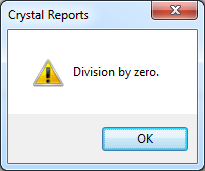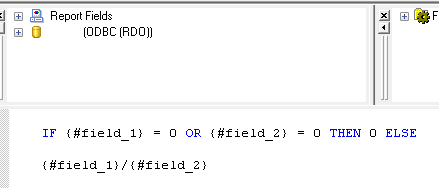Crystal Reports – How to fix ‘Division by zero’ error

Problem

When running a Crystal Report you receive the following error message

Division by zero.Solution

Before describing how to work around this issue a quick explanation.

When you see this message it means a formula in the report is attempting to calculate a value using a field that has either a NULL or 0 value. You cannot divide by NULL or 0 – it is mathematical non-sense.

For example

1 / 0 =  mathematical non-sense

To fix the issue you need to find the formula that’s causing the issue and add a check at the start to say ‘if value is 0 then return 0 else do calculation’.

For example

IF {#field_1} = 0 OR {#field_2} = 0 THEN 0 ELSE
{#field_1}/{#field_2}One comment on “Crystal Reports – How to fix ‘Division by zero’ error”

1. Jane says:

helped a lot. thanks